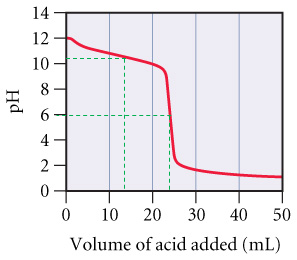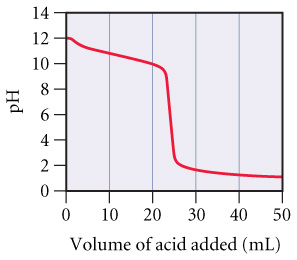# Problem: Consider the following curve for the titration of a weak base with a strong acid and answer each of the following questions.At what volume of added acid does pH = 14 – pKb?

###### FREE Expert Solution

At 1/2 equivalence point: pH = pKa

pKa + pKb = 14

So we know that at 1/2 equivalence point:

pH = 14 – pKb

From the graph:93% (460 ratings)###### Problem Details

Consider the following curve for the titration of a weak base with a strong acid and answer each of the following questions.At what volume of added acid does pH = 14 – pKb?